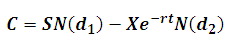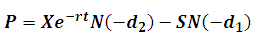# Black-Scholes Option Pricing Model

Black-Scholes Option Pricing Model is the most popularly used model for pricing financial options.

The formula was published by Fischer Black and Myron Scholes in 1973 in their groundbreaking paper “The Pricing of Options and Corporate Liabilities”.

Using the Black-Scholes model, the price of a call option is calculated using the following formula:Where: C is the price of the call option S is the price of the underlying stock X is the option exercise price r is the risk-free interest rate T is the current time until expiration N() is the area under the normal curve d1 =  [ ln(S/X) + (r + σ2/2) T ] / σ T1/2 d2 =  d1 - σ T1/2

Similarly, the price of the put option is:Assumptions

The Black-Scholes model has the following assumptions:

1. Options can be exercised only at expiration 2. Risk-free rate and volatility remain constant over the life of the option 3. The underlying stock does not pay dividends

Example:

Consider a European Call Option on 100 shares of stock XYZ with the following details: Strike price: USD 60 Time to maturity: 0.5 years. Spot price: USD 50 Volatility: 30% Risk free interest rate: 3%

Using the Black-Scholes formula, the price of the call option will be $1.4. Since the option is on 100 shares, the total value will be$140.

# R Programming Bundle: 25% OFF

Get our R Programming - Data Science for Finance Bundle for just $29$39.
Get it now for just \$29